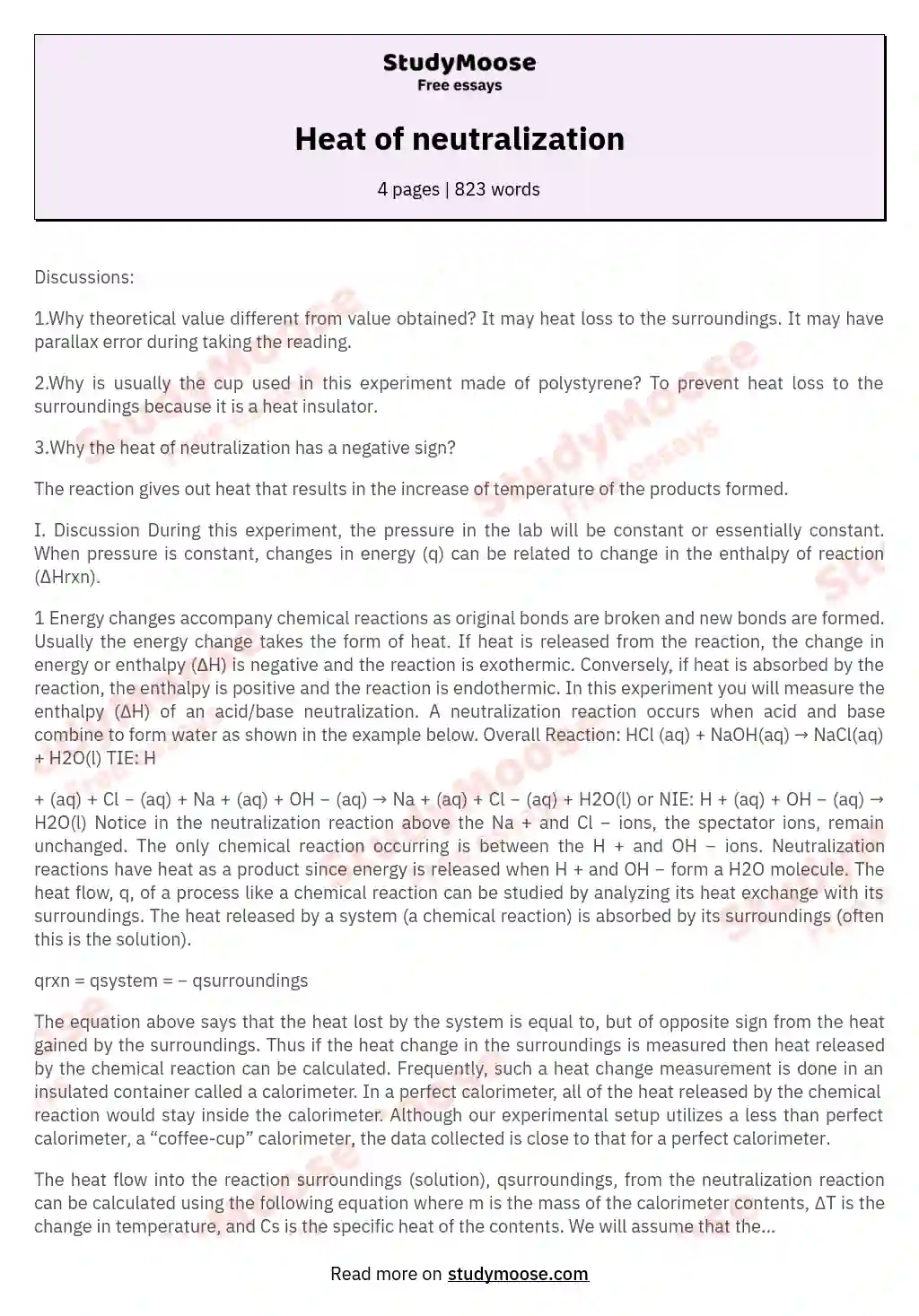# Heat of neutralization

Categories: Heat

Discussions:

1.Why theoretical value different from value obtained?
It may heat loss to the surroundings.
It may have parallax error during taking the reading.

2.Why is usually the cup used in this experiment made of polystyrene? To prevent heat loss to the surroundings because it is a heat insulator.

3.Why the heat of neutralization has a negative sign?

The reaction gives out heat that results in the increase of temperature of the products formed.

I. Discussion During this experiment, the pressure in the lab will be constant or essentially constant.

When pressure is constant, changes in energy (q) can be related to change in the enthalpy of reaction (ΔHrxn).

1 Energy changes accompany chemical reactions as original bonds are broken and new bonds are formed. Usually the energy change takes the form of heat. If heat is released from the reaction, the change in energy or enthalpy (∆H) is negative and the reaction is exothermic. Conversely, if heat is absorbed by the reaction, the enthalpy is positive and the reaction is endothermic.

Get quality help nowRhizManVerified writer

Proficient in: Heat4.9 (247)

“ Rhizman is absolutely amazing at what he does . I highly recommend him if you need an assignment done ”+84 relevant experts are online

In this experiment you will measure the enthalpy (∆H) of an acid/base neutralization. A neutralization reaction occurs when acid and base combine to form water as shown in the example below. Overall Reaction: HCl (aq) + NaOH(aq) → NaCl(aq) + H2O(l) TIE: H

+
(aq) + Cl

(aq) + Na
+
(aq) + OH

(aq) → Na
+
(aq) + Cl

(aq) + H2O(l)
or
NIE: H
+
(aq) + OH

(aq) → H2O(l)
Notice in the neutralization reaction above the Na
+
and Cl

ions, the spectator ions, remain unchanged.

Get to Know The Price Estimate For Your Paper
Topic
Number of pages
Email Invalid email

You won’t be charged yet!

The only chemical reaction occurring is between the H
+
and OH

ions. Neutralization reactions have
heat as a product since energy is released when H
+
and OH

form a H2O molecule.
The heat flow, q, of a process like a chemical reaction can be studied by analyzing its heat exchange with its surroundings. The heat released by a system (a chemical reaction) is absorbed by its surroundings (often this is the solution).

qrxn = qsystem = − qsurroundings

The equation above says that the heat lost by the system is equal to, but of opposite sign from the heat gained by the surroundings. Thus if the heat change in the surroundings is measured then heat released by the chemical reaction can be calculated. Frequently, such a heat change measurement is done in an insulated container called a calorimeter. In a perfect calorimeter, all of the heat released by the chemical reaction would stay inside the calorimeter. Although our experimental setup utilizes a less than perfect calorimeter, a “coffee-cup” calorimeter, the data collected is close to that for a perfect calorimeter.

The heat flow into the reaction surroundings (solution), qsurroundings, from the neutralization reaction can be calculated using the following equation where m is the mass of the calorimeter contents, ∆T is the change in temperature, and Cs is the specific heat of the contents. We will assume that the solution in the calorimeter has the same physical properties as water, specifically that Cs = 4.184 J/g•°C. qsurroundings = m ∆T Cs

In contrast when volume is constant, changes in energy (q) can be related to changes in the internal energy, ΔErxn.2 In this experiment the neutralization of sodium hydroxide (a strong base) with hydrochloric acid (a strong acid) and acetic acid (a weak acid) will be investigated. The base is present in slight excess and, therefore, the acid is the limiting reagent and determines the number of moles of acid and base reacting. Therefore, the heat flow from the reaction is:

qrxn = ∆Hrxn (mol acid

II. Objectives

1. To determine the enthalpy of neutralization of a strong base with a strong acid. 2. To determine the enthalpy of neutralization of a strong base with a weak acid. 3. To use Hess’ Law to determine the enthalpy of dissociation of the weak acid.

III. Procedure – This lab is done is pairs.

A. Preparation of Solutions

1. Each lab bench will need make the 0.5000 M acid solutions needed for this lab by diluting 1.000 M stock solutions of HCl and acetic acid. Read the label on the container to obtain the exact molarity of the acid solutions. It will be very close to 1.000M. Lab groups at each bench will share these solutions.

2. Each pair of students will need at least 120 mL of each acid. Accounting for errors and/or extra trials, a total volume of 500 mL of the 0.5000 M acids will be enough for 3 pairs of students.

3. The equipment available for the dilution includes 250.00 and 500.00 mL volumetric flasks. Your instructor will demonstrate how to perform the dilution. (Although volumetric glassware is not commonly used to make quantitative dilutions, the process is appropriate for the solutions used in this lab.)

Recall the formula for dilution calculations:
M1V1 = M2V2
For this dilution:
M1 = 250.00 mL
V1 = Molarity of the stock acid solution(known)
M2, = Molarity of the dilute acid solution(solve for this – should be close to 0.5000 M) V2 = 500.00 mL